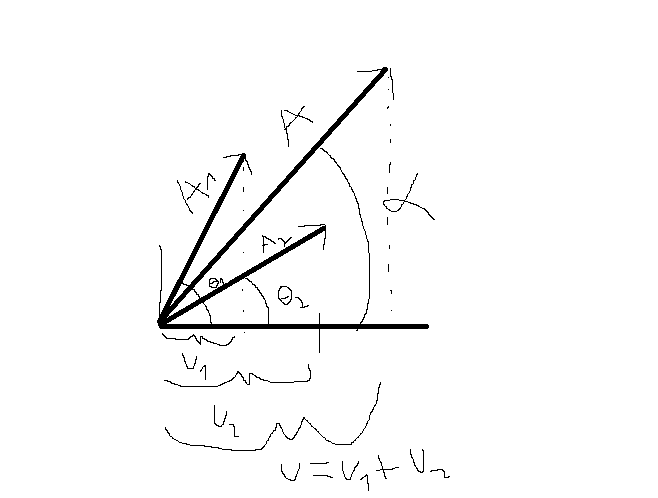# Two wave superposition with different wavelength , amplitude and frequ

## Homework Statement

Hi

Two wave superposition with different wavelength , amplitude and frequency

u1(x,t)=A1cos(k1x+w1t)
u2(x,t)=A2cos(k2x+w2t)

a)Show that an amplitude modulation is obtained

## Homework Equations

No relevant equations

## The Attempt at a Solution

u=u1+u2=A1cos(k1x+w1t)+A2cos(k2x+w2t)

and now i dont know what to do, is there any way to relate A1 and A2

I searched in the internet and I found Fresnell method which can solve it for two waves of the tipe:
u1=u2=A1cos(w1t)
u2=A1cos(w2t)=A1cos(w1t+phi)

but in my case I dont think I can do that because there are 2 variables in the cos

Its my first post so thanks
pd: sorry for my bad english

vela
Staff Emeritus
Homework Helper
Try this: Draw a right triangle with the length of one leg equal to ##A_1##, the length of the other leg equal to ##A_2##, and with hypotenuse equal to ##\sqrt{A_1^2+A_2^2}##. Then express ##A_1## and ##A_2## in terms of ##A## and an angle ##\theta##, appropriately defined. That might get it into a form you can more easily work with.

I'm just making a suggestion. I haven't actually worked the problem out, so the suggestion may not turn out to be useful.

Expresing in terms of A=√A21+A22

I arrive to the expresion

u(x,t)=(A/sinθ)(tan(θ)cos(k1x+w1t)+cos(k2x+w2t)) I dont see how it can help :/ but thanks

Maybe I can prove that theres a Amplitude modulation without merging the cosI think i found a way to solve it so if i make two vectors where A1 and A2 is the module of each vector I just have to sum them and then find the angle of the resulting vector.

Where θ1=k1x-w1t
θ2=k2x-w2t
u1=A1cosθ1
u2=A2cosθ2

And alfa=(θ1-θ2)/2+θ2=θ1+θ2/2